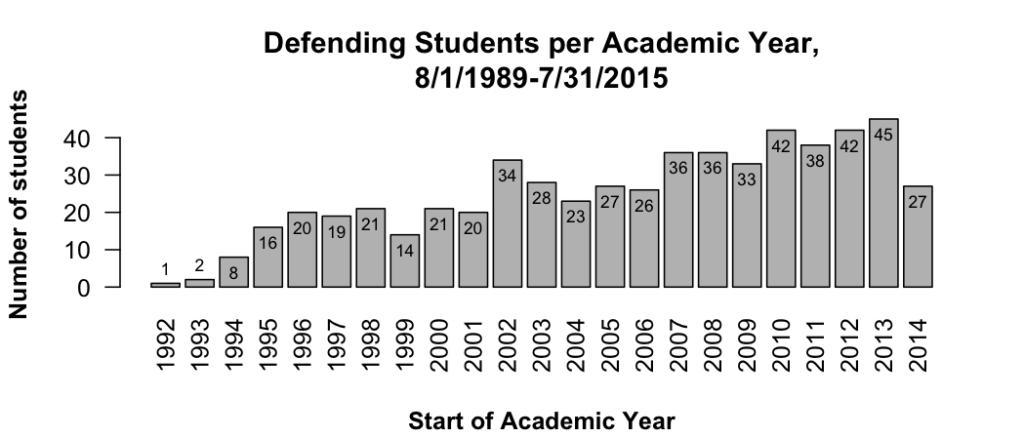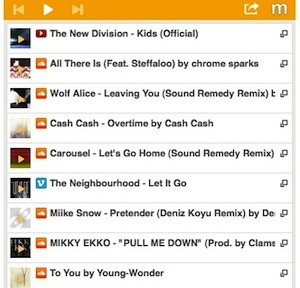# Appending Python’s Path

Find the directory that Python searchers for path additions:
`python -c 'import site; site._script()' --user-site`

In my case, it was:
~/Library/Python/2.7/lib/python/site-packages

Create a text file there with an extension “.pth” and add directories. My file looks something like:

~/Library/Python/2.7/lib/python/site-packages/mc_custom.pth:
``` # Custom additions on 5/27/16 /Users/mclaffey/Documents/my_modules ```

Source

# Non-ggplot bar graph with data labels

``` p = function() { x = gd\$year y = gd\$defended_n bp = barplot( y, names.arg = x, las = 2 # make years vertical by making all ticks perpendicular ) # title title(main="Defending Students per Academic Year,\n8/1/1989-7/31/2015") # x axis title(xlab="Start of Academic Year", font.lab=2, line=4) # y axis title(ylab="Number of students", font.lab=2) # data data labels label_height = y - 4 label_height = label_height + 8 # the first one is too low, bump it up label_height = label_height + 8 # the second one is too low, bump it up text(bp, label_height, y, cex=.7) } ```# Side-by-side bars with error bars

Simple side-by-side (no error bars):
http://stackoverflow.com/a/25070645

# Hiding the legend

``` # Remove legend for a particular aesthetic (fill) bp + guides(fill=FALSE)```

``` # It can also be done when specifying the scale bp + scale_fill_discrete(guide=FALSE) ```

```# This removes all legends bp + theme(legend.position="none") ```

# Rotating Axis Labels

http://stackoverflow.com/questions/1330989/rotating-and-spacing-axis-labels-in-ggplot2

Also makes the text smaller (assuming you are rotating because of long labels).

``` q + theme(axis.text.x = element_text(angle = 90, hjust = 1, size=20)) ```

# Percent labels for axis

http://stackoverflow.com/questions/27433798/how-to-change-y-axis-range-to-percent-from-number-in-barplot-with-r

``` library(scales) myplot <- qplot(as.factor(x), y, geom="bar") myplot + scale_y_continuous(labels=percent) ```

# Dodging

Use position='dodge' when you are okay with the default behavior.

Use position=position_dodge(width = 0.90, height=2) when you want to control the behavior.

# Zooming

If you use scale_y_XXX(limits=c(50, 100)), any graph object that falls outside that window at all will be completely removed from the figure, even if a different portion falls within the limits. To avoid this, use the following to 'zoom' in the figure, without changing what objects are included.

``` p + coord_cartesian(xlim = c(325, 500)) ```

# ggplot's text justification

http://stackoverflow.com/questions/7263849/what-do-hjust-and-vjust-do-when-making-a-plot-using-ggplot

Think of hjust as the percentage of the text's horizontal width that is left of the anchor point. So 0 means 0% of the text is left of the anchor point, so this is left justified. 0.5 means 50% of the text is left of the anchor point, so the text is right justified. 1 is 100% is right justified.

vjust is the percent of height **below** the anchor.

A way to remember this is that for an anchor point at 0,0, and with hjust=vjust=0, the text would appear properly in upper right quadrant from the origin.

Note that if the text is rotated, the justification is applied before the rotation. For example, text with hjust=0 and vjust=1 is right of and below the anchor point. If it is then rotated 90 degrees counterclockwise, it ends up right of but above the anchor point.

# Axis label spacing

The default theme doesn't have enough space between axis text and tick marks.

# Boilerplate at top of iPython notebook

``` # pandas import pandas as pd pd.set_option('display.max_columns', None) pd.set_option('display.max_colwidth', -1) # plotting import matplotlib.pyplot as plt plt.style.use('ggplot') def plt_remove_offset(): plt.gca().ticklabel_format(useOffset=False); # custom libraries import scripps import dfx # R %load_ext rpy2.ipython %R source("../r/plot_help.R"); # setup output directories scripps.setup_output_folder() ```

# plot_help.R

``` require(ggplot2, quietly=TRUE) require(scales, quietly=TRUE) save_and_display_figure = function(fig_path, fig_object=p) { # fig_path should be a string, as passed to png(file) # p should be either: # - a ggplot object, such as p = ggplot(data) + geom_bars() + ... # - a function that produces a plot, such as p=function(){hist(c(1, 1, 2, 5, 5))} # usage in iPython: # %%R -w 7 -h 5 -u in # p = ggplot(data) + geom_bars() + ... # save_and_display_figure("figures/some_figure") display_fig = function() { if ('ggplot' %in% class(fig_object)) { #cat("Displaying ggplot figure\n") print(fig_object) } else { #cat("Calling figure code\n") fig_object() } } # use the current display size for the size of the image file windows_size_inches = dev.size('in') #cat("Current window size:", windows_size_inches, "wide inches by", windows_size_inches, "high") # print to file png(file=fig_path, width=windows_size_inches, height=windows_size_inches, units='in', res=150) display_fig() dev.off(); # print to screen display_fig() } theme_mcSimple <- function(base_size=14, base_family="", legend_title=TRUE, legend=TRUE, axes_lines=TRUE, horz_gridlines=TRUE, x_axis_font_size=NA) { # start with basic black and white theme, which specified font mc_theme = theme_bw(base_size=base_size, base_family=base_family) # figure is white background with optional horizontal gridlines if (horz_gridlines) { mc_theme = mc_theme + theme( panel.grid.minor = element_blank(), panel.grid.major.x = element_blank(), panel.grid.major.y = element_line(colour = "grey") ) } else { mc_theme = mc_theme + theme(panel.grid = element_blank()) } # turn off border, and maybe turn on left/bottom axes mc_theme = mc_theme + theme(panel.border = element_blank()) if ( axes_lines ) { mc_theme = mc_theme + theme(axis.line = element_line(colour = "black")) } # space between axis title and ticks mc_theme = mc_theme + theme( plot.title = element_text(margin = margin(b = base_size)), axis.title.y=element_text(margin=margin(0,20,0,0)), axis.title.x=element_text(margin=margin(20,0,0,0)) ) # visibility of legend and legend title if ( ! legend ) { mc_theme = mc_theme + theme(legend.position="none") } else if ( ! legend_title) { mc_theme = mc_theme + theme(legend.title=element_blank()) } # x-axis tick font size if (! is.na(x_axis_font_size)) { mc_theme = mc_theme + theme(axis.text.x = element_text(size=x_axis_font_size)) } # hide all ticks mc_theme = mc_theme + theme(axis.ticks = element_blank()) return(mc_theme) } ```

# Complete ggplot theme

Below is the source code for the ggplot theme_grey, as found here. This is useful when creating your own customized theme, as it covers most of the available elements and how to adjust them.

``````
theme_grey <- function(base_size = 11, base_family = "") {
half_line <- base_size / 2

theme(
# Elements in this first block aren't used directly, but are inherited
# by others
line =               element_line(colour = "black", size = 0.5, linetype = 1,
lineend = "butt"),
rect =               element_rect(fill = "white", colour = "black",
size = 0.5, linetype = 1),
text =               element_text(
family = base_family, face = "plain",
colour = "black", size = base_size,
lineheight = 0.9, hjust = 0.5, vjust = 0.5, angle = 0,
margin = margin(), debug = FALSE
),

axis.line =          element_blank(),
axis.text =          element_text(size = rel(0.8), colour = "grey30"),
axis.text.x =        element_text(margin = margin(t = 0.8 * half_line / 2), vjust = 1),
axis.text.y =        element_text(margin = margin(r = 0.8 * half_line / 2), hjust = 1),
axis.ticks =         element_line(colour = "grey20"),
axis.ticks.length =  unit(half_line / 2, "pt"),
axis.title.x =       element_text(
margin = margin(t = 0.8 * half_line, b = 0.8 * half_line / 2)
),
axis.title.y =       element_text(
angle = 90,
margin = margin(r = 0.8 * half_line, l = 0.8 * half_line / 2)
),

legend.background =  element_rect(colour = NA),
legend.margin =      unit(0.2, "cm"),
legend.key =         element_rect(fill = "grey95", colour = "white"),
legend.key.size =    unit(1.2, "lines"),
legend.key.height =  NULL,
legend.key.width =   NULL,
legend.text =        element_text(size = rel(0.8)),
legend.text.align =  NULL,
legend.title =       element_text(hjust = 0),
legend.title.align = NULL,
legend.position =    "right",
legend.direction =   NULL,
legend.justification = "center",
legend.box =         NULL,

panel.background =   element_rect(fill = "grey92", colour = NA),
panel.border =       element_blank(),
panel.grid.major =   element_line(colour = "white"),
panel.grid.minor =   element_line(colour = "white", size = 0.25),
panel.margin =       unit(half_line, "pt"),
panel.margin.x =     NULL,
panel.margin.y =     NULL,
panel.ontop    =     FALSE,

strip.background =   element_rect(fill = "grey85", colour = NA),
strip.text =         element_text(colour = "grey10", size = rel(0.8)),
strip.text.x =       element_text(margin = margin(t = half_line, b = half_line)),
strip.text.y =       element_text(angle = -90, margin = margin(l = half_line, r = half_line)),

plot.background =    element_rect(colour = "white"),
plot.title =         element_text(
size = rel(1.2),
margin = margin(b = half_line * 1.2)
),
plot.margin =        margin(half_line, half_line, half_line, half_line),

complete = TRUE
)
}
```
```

# Goal

Set up a hard drive that is bootable to either OS X 10.6 or Ubuntu, but with a shared user partition so that user data is accessible regardless of which operating system is booted into.

# Convert flv to mov (and deal with ffmpeg’s “incorrect parameters” error)

Goal: Convert a Flash .flv file to .mov on OS X.

I’ve used ffmpeg before and knew this was a good tool for the job, but hadn’t used it in awhile and wasn’t sure I even had the binary installed.# Embedded playlist on a web page

I wanted to be able to share playlists of music on my web site. Ideally I wouldn’t have to host the music itself but could instead point to different sources like youtube, soundcloud, ex.fm. After some research, Minilogs seemed to be the best solution.

# December 2012 Music

Every month I make a playlist of random songs I come across (mostly from hype machine).

Below is December’s playlist, which you can play directly with Minilogs‘s embedded player.

Here’s a post I wrote about finding the right embedded playlist solution.

Click on the play buttonbelow: